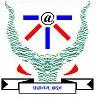Ross and UNAGI
Tag(s):

Easy-Medium

Problem
Editorial
AnalyticsRachel and Phoebe are sick of Ross trying to teach them the importance of UNAGI and decide to give him a problem to keep him busy . Ross asks for your help to solve the problem :

You are given Q queries containing two integers a,b . Your task is simple. Calculate sum of prime factors of
($a^{b^{c}}$ + 1)%m where m is $10^{6} + 3$

Input :

First line contains single integer Q .
Next Q lines contain three spaced integers a,b,c.

Output :

For each query output the answer in a new line.

Constraints :

$1 \leq Q \leq 10^{6}$

$0 \leq a,b \leq 10^{9}$

$0 \leq c \leq 10^{18}$

Problem Setter - Vaibhav Bansal

SAMPLE INPUT
2
2 3 2
3 2 2
SAMPLE OUTPUT
28
43
Time Limit: 3.0 sec(s) for each input file.
Memory Limit: 256 MB
Source Limit: 1024 KB
Marking Scheme: Marks are awarded when all the testcases pass.
Allowed Languages: Bash, C, C++, C++14, Clojure, C#, D, Erlang, F#, Go, Groovy, Haskell, Java, Java 8, JavaScript(Rhino), JavaScript(Node.js), Julia, Kotlin, Lisp, Lisp (SBCL), Lua, Objective-C, OCaml, Octave, Pascal, Perl, PHP, Python, Python 3, R(RScript), Racket, Ruby, Rust, Scala, Swift, Swift-4.1, TypeScript, Visual Basic

CODE EDITOR

Initializing Code Editor...

This Problem was Asked inChallenge Name

Trihacker - IIITA (Mirror)

OTHER PROBLEMS OF THIS CHALLENGE
• Math > Combinatorics